x2
Introduction
( )
Types of Arrangements
=
Two More Methods
=
Some Applications

# Infinite Series

## Infinite Series

In all of our previous examples, the series all had a finite number of terms, i.e. the counters all had a starting number and an ending number. If we take off the upper number and let the series keep going indefinitely, we get what's called an "infinite series". For example

$$\sum_{i=1}^\infty \frac{1}{i} = \frac{1}{1}+\frac{1}{2}+\frac{1}{3}+\frac{1}{4}+...$$ $$\sum_{i=0}^\infty 2^i = 1+4+6+9+16+...$$ $$\sum_{i=0}^\infty \frac{1}{2^i} = \frac{1}{1} + \frac{1}{4} + \frac{1}{8} + ...$$

At this point, you might be thinking, "How can we talk about adding up infinitely many numbers? That can't be possible." That's a valid point and the first thing I should clarify is that when we talk about "the sum of an infinite series", we aren't talking about adding up all the numbers like we did with finite series. To see how we define this kind of sum, take a look at the last series above,

This is just the geometric series with r = 1/2 that we saw in the last section. The table below has the results that we get from adding up the first few terms of the series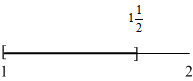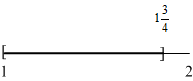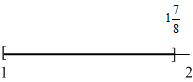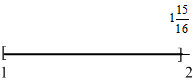The diagrams in the right hand column illustrate what's going on as the number of terms increases. Notice how every time we add a term, the total gets a little bit closer to 2 but never goes past it. That's what we mean when we say that an infinite series equals a number: As the value of i gets bigger, the sum gets really close to the number but never becomes greater than the number.

If all this talk about "really close to" seems kind of vague, you're right. A precise definition of what we mean by "close" belongs in a calculus class so we're going to have to put it off for later. For now, what you should understand is that the "sum" of an infinite sequence is the smallest upper limit on the "partial sums", i.e. the sums that you get from adding up the beginning part of of the series.

## Infinite Geometric Series

Finding that "smallest upper limit" for a specific series can be a tricky business. In the special case of our geometric series, there's a simple formula that you can use.

Sum of an Infinite Geometric Series
$\sum_{i=0}^\infty ar^i = \frac{a}{1-r}$ when |r| < 1.

# Example 1 - Infinite Geometric Series

Find the sum of $\sum_{i=0}^\infty 3 \left(\frac{1}{3}\right)^i$.

That's an infinite geometric series with a = 3 and r = 1/3. Substituting those values into our formula gives

$$\sum_{i=0}^\infty 3 \left(\frac{1}{3}\right)^i = \frac{3}{1-\frac{1}{3}} = \frac{3}{\frac{2}{3}} = \frac{9}{2}$$

# Example 2 - Infinite Geometric Series

Find the sum of $1 - \frac{1}{2} + \frac{1}{4} - \frac{1}{8} + \frac{1}{16} - ...$.

This is an infinite geometric series where a = 1 and r = -1/2. Substituting those values into our formula gives

$$1 - \frac{1}{2} + \frac{1}{4} - \frac{1}{8} + \frac{1}{16} - ... = \frac{1}{1-\frac{-1}{2}} = \frac{1}{1+\frac{1}{2}} = \frac{1}{\frac{3}{2}} = \frac{2}{3}$$

Keep in mind that our formula has an important limitation: |r| < 1. What happens if |r| ≥ 1? In those cases, the series doesn't have a sum.2 This is an important point that you need to be aware of: Not all infinite series have a sum or "converge" to a value.

# Example 3 - Decimals vs. Fractions

Write .444... as a fraction.

To do this, we have to think a little creatively about what the decimal version means. First, I'll write it out with a few more digits

.4444...

Here's the creative part: I'm going to rewrite the decimal as a sum.

.4 + .04 + .004 + .0004 + .00004 + ...

.4 + .4 (.1) + .4(.01) + .4(.0001) + ...

.4 + .4 (.1) + .4 (.1)2 + .4(.1)3 + ...

Now I've rewritten the decimal as an infinite geometric series where a = .4 and r = .1. That means that

$$.444...=\sum_{i=0}^\infty .4 (.1)^i = \frac{.4}{1-.1} = \frac{.4}{.9} = \frac{4}{9}$$

## Approximations with Infinite Series

Suppose I asked you to find the value of e1.8. You'd probably just take out your calculator, press a few buttons and give me the answer. That works great today but what would you do without your calculator? There's a whole branch of math called approximation theory that studies how you can take hard to calculate functions like f(x) = ex and approximate them using functions that are easier to work with. Many of these approximations end up being infinite series. For example,

$$f(x)=e^x = \sum_{i=0}^{\infty} \frac{x^i){i!}$$

What makes a formula like this useful is that it gives you a way to calculate values for the function that just involve basic arithmetic, i.e. multiplication, division, addition and subtraction.

# Example 4 - Approximating Values

Use the first five terms of the series form of ex to find an approximate value for e2.

The first five terms of the series are

Substituting 2 for x gives us

$$e^2 \approx 1 + 2 + \frac{2^2}{2!} + \frac{2^3}{3!} + \frac{2^4}{4!}$$ $$e^2 \approx 1 + 2 + 2 + 1.33 + .66$$ $$e^2 \approx 6.99$$

# Example 5 - Approximating Values

Use the first six terms of the series form of ex to find an approximate value for e2.

The first five terms of the series are

Substituting 2 for x gives us

$$e^2 \approx 1 + 2 + \frac{2^2}{2!} + \frac{2^3}{3!} + \frac{2^4}{4!} + \frac{2^5}{5!}$$ $$e^2 \approx 1 + 2 + 2 + 1.33 + .66 + .266$$ $$e^2 \approx 7.265$$

When we were looking at the formula for an infinite geometric series, it had a limitation. It only worked for values of r that were between -1 and 1. These approximation series, called Taylor series after Brook Taylor, an 18th century mathematician who came up with a formula for deriving them, also often have a limited range in which they're accurate.

# Exercises

### Exercises= show solution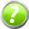= get hint

n
1. $\sum_{i=0}^\infty 4\left(\frac{2}{5}\right)^i$
2. $\sum_{i=0}^\infty \left(\frac{1}{8}\right)^i$
3. $\sum_{i=0}^\infty -2\left(\frac{1}{3}\right)^i$
4. $\sum_{i=0}^\infty 8\left(\frac{-3}{5}\right)^i$
5. $\sum_{i=0}^\infty 6(1)^i$
6. $\sum_{i=5}^\infty 2\left(\frac{9}{10}\right)^i$
7. $\sum_{i=0}^\infty 1.6(-0.3)^i$
8. $\sum_{i=2}^\infty 2\left(\frac{-5}{3}\right)^i$
9. $\sum_{i=2}^\infty 2(0.28)^i$
10. 0.88...
11. 0.33...
12. 0.4343...

### Analysis

1. 0.0808...
2. 0.5111...
3. 0.99...
4. Show that the series $\sum_{i=1}^\infty i = 1 + 2 + 3 + 4 + ...$ doesn't converge. (Hint: Show that no matter what number you pick to be the sum, the series eventually gets bigger than that number.)

5. The Taylor series for the logarithmic function is

$$\sum_{i=1}^\infty(-1)^{i+1} \frac{(x-1)^i}{i}$$

Use the Taylor series with four terms to calculate ln(1.4).

6. Use the Taylor series with five terms and the series with six terms to calculate ln(1.4). Compare the results to the value of ln(1.4) that you get from using a calculator. Discuss the impact of adding additional terms in terms of your results.
7. Use the Taylor series for the logarithmic function to calculate ln(2) using the first four, five and six terms. Compare the results to the value that you get from using a calculator. How does the accuracy of these results compare with the accuracy that you got in the previous question?3

2 If it bothers you to talk about "a sum" when this isn't really the sum of a list of numbers, we also use the word "converge" in these situations, e.g. we could also say that the series in Example 1 converges to 9/2.

3 What you're seeing here is a common problem with Taylor series. Even for a value where the series converges, the number of terms that it takes to get a good result can be different for different values of x.